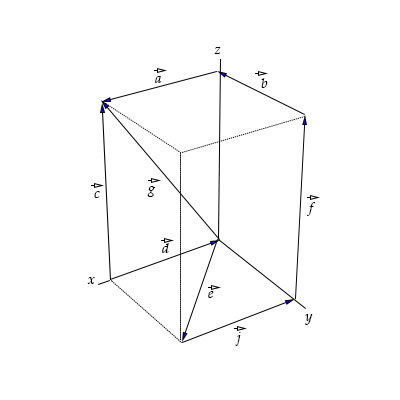# The figure shows a rectangular box in three dimensional space that contains several vectors. (The...

## Question:

The figure shows a rectangular box in three dimensional space that contains several vectors. (The vector {eq}\vec c {/eq} is in the {eq}xz {/eq} plane, the vector {eq}\vec e {/eq} is in the {eq}xy {/eq} plane). Are the following statements true or false?

a. {eq}\vec a = \vec d {/eq}

b. {eq}\vec c = \vec f {/eq}

c. {eq}\vec d= \vec g-\vec c {/eq}

d. {eq}\vec g = \vec f +\vec a {/eq}

e. {eq}\vec a= -\vec b {/eq}

f. {eq}\vec e= \vec a - \vec b {/eq}## Vectors

Geometrically, two vectors can be added by the triangle rule if the two vectors have the same initial point,

then the resultant vector is the vector connecting the terminal points.

Two vectors are equal if they have the same direction and equal lengths, therefore there area infinitely many equal vectors, with the reference vector given by the vector with the initial point at the origin.

a. The statement {eq}\boxed{ \vec a = \vec d \text{ is FALSE }} {/eq} because they have opposite directions, {eq}\vec a {/eq} is in the direction of positive x, while {eq}\vec d {/eq} is in the direction of negative x axis.

b. The statement {eq}\boxed{ \vec c = \vec f \text{ is TRUE }} {/eq}

because they have the same directions, in the direction of positive z axis and same length,

the height of the rectangular box.

c. The statement {eq}\boxed{ \vec d = \vec g-\vec c \text{ is FALSE }} {/eq} because by the triangle rule {eq}\vec c= \vec d+\vec g\implies \vec d= -\vec g+\vec c\neq \vec g-\vec c. {/eq}

d. The statement {eq}\boxed{ \vec g = \vec f+\vec a \text{ is TRUE }} {/eq}

because the reference vector of {eq}\vec f {/eq} (given by the vector with the initial point at the origin and direction in the positive direction of z),

added with the vector {eq}\vec a {/eq}

satisfies the triangle rule to obtain the vector {eq}\vec g: \vec g= \vec f+\vec a. {/eq}

e. The statement {eq}\boxed{ \vec a = -\vec b \text{ is FALSE }} {/eq}

because their directions are not parallel, they are perpendicular.